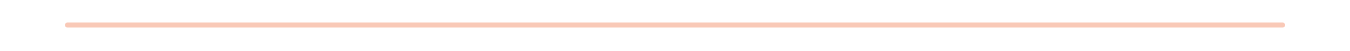# Deep learning is accessible to you!

## You don’t need to be a mathematics graduate to understand and apply deep learning.Deep learning seems like a daunting topic with its complex maths and advanced model architectures. But once things are broken down into digestable pieces, there is nothing to be scared of!

This course will provide you with just enough theory to understand:

• what deep learning is
• its building blocks
• architecture of latest algorithms

So that we can together:

• apply the most common models on interesting problems
• get experience building DL models
• deploy the models to be used in real-life

Module 1: Introduction to Deep Learning
• What is Deep Learning?
• What is the difference of Machine Learning and Deep Learning?
• What are common techniques of Deep Learning?
• Applications of Deep Learning in the real world.
Module 2: Building Blocks of Deep Learning
• Introduction to Neural Networks
• Meet the parameters: Bias and Weight
• Activation functions
• Forwards propagation
• Backwards propagation
Exercise 1: Build your own NN
• Introduction to Tensorflow and Keras
• Importing libraries
• Datasets and how to use them
• Setting up your first Neural Network
Module 3: Training Deep Learning Models
• Setting up hyperparameters
• Dealing with over/underfitting
• Lowering computation time
• Hyperparameter tuning
• Evaluating the model
Exercise 2: Deep NNs at Work
• Setting up a complex neural network with many layers
• How to make key decisions in practice
• How to identify and solve issues in your network
Module 4: Data issues
• Types of data: structured and unstructured
• Where to find quality datasets
• Where to find big datasets
• Dealing with a lack of data
Module 5: Convolutional Neural Networks
• How do CNNs work?
• Understanding CNNs layer by layer
• Common CNN architectures
Exercise 3: Object Detection Using CNNs
• Plan and implement an object detection model
• Set up a web app to showcase the model's capabilities
Module 6: Recurrent Neural Networks
• How do RNNs work?
• Common RNN architectures and components
• A closer look at LSTMs
• How to build better models with Attention
Exercise 4: Text generation with RNNs
• How to use pre-trained models as base
• Implementing a text generating model